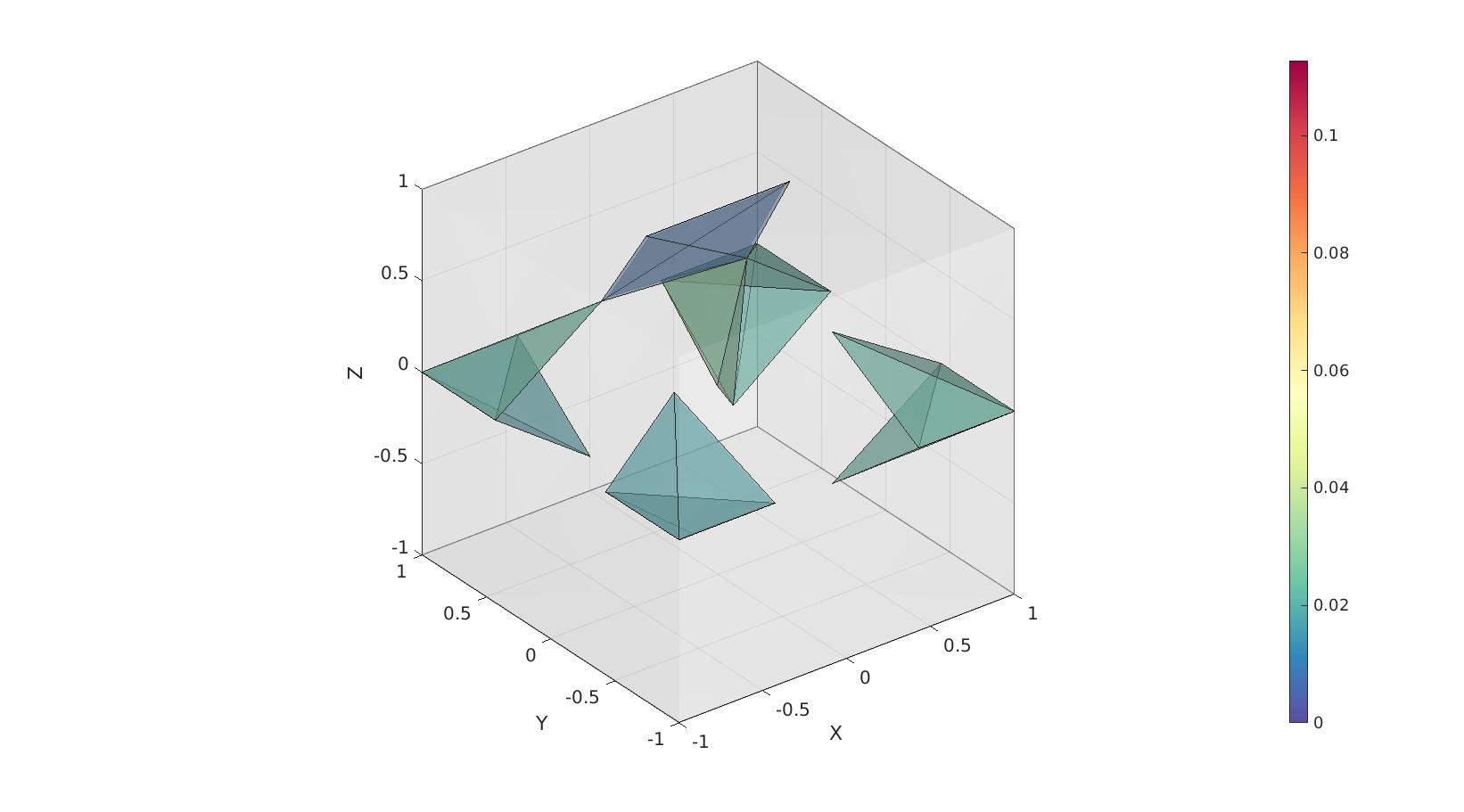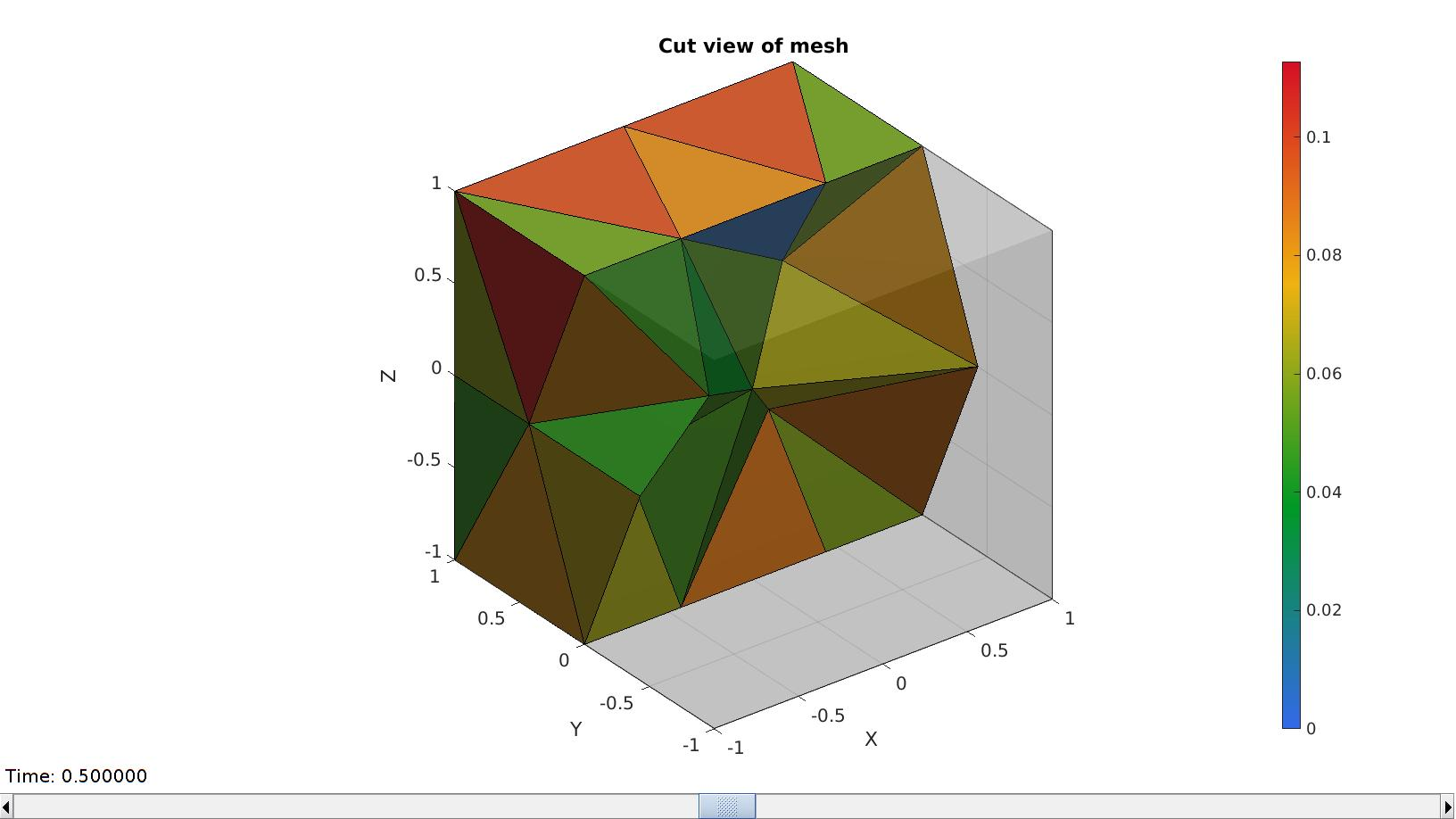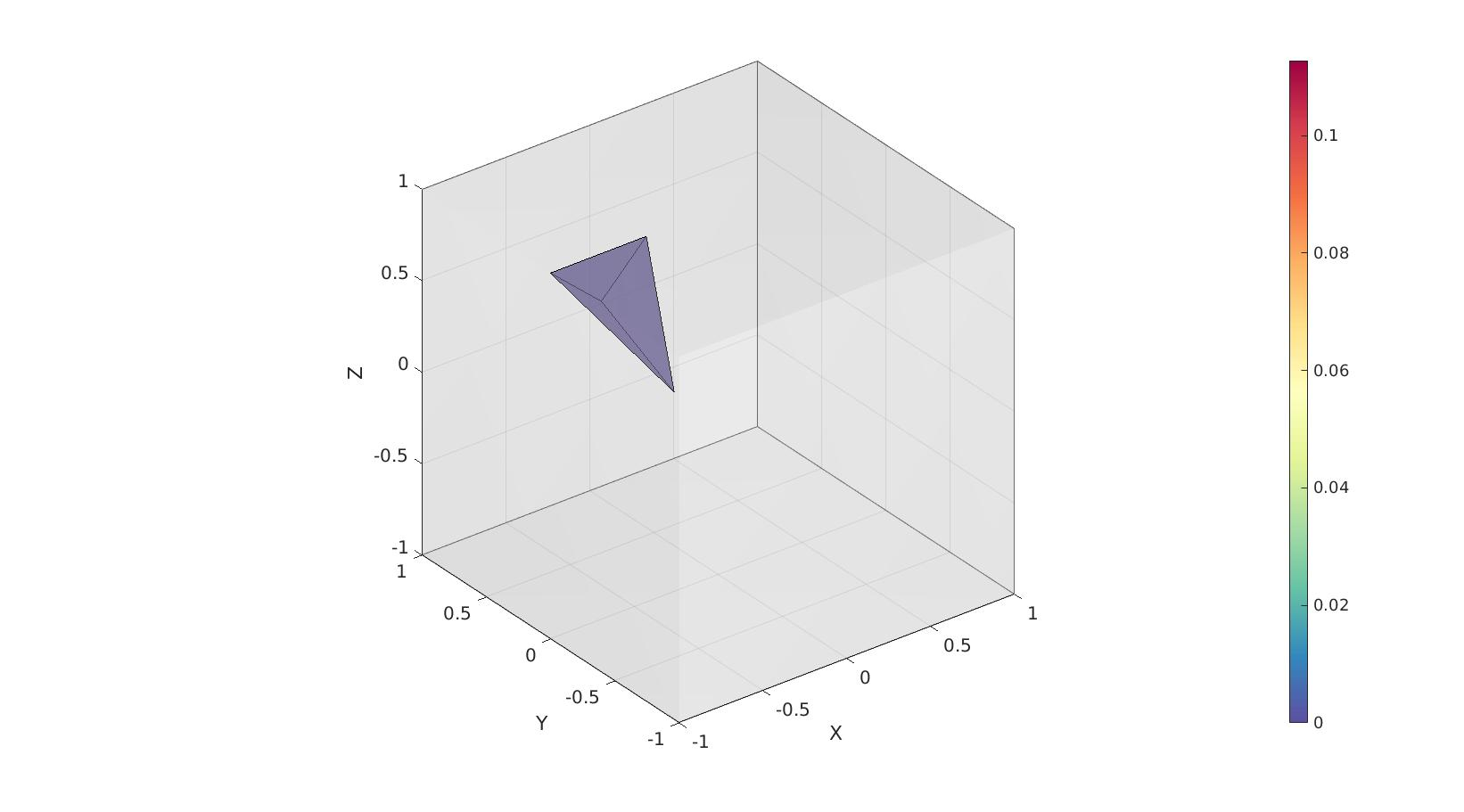# tetVol

Below is a demonstration of the features of the tetVol function

## Contents

```clear; close all; clc;
```

## Syntax

[VE,L]=tetVol(E,V);

## Description

This function computes tetrahedral element volumes. The input is the element description (E) and the nodes (V). The output is the element volumes (always positive) and a logic denoting wheter the element appears to be valid (1) or inverted (0).

## Examples

Plot settings

```cMap=gjet(250);
faceAlpha1=1;
faceAlpha2=0.65;
edgeColor1='none';
edgeColor2='none';
fontSize=15;
```

## Example: Computing the volume of tetrahedral elements

Create example geometry

```% Creating a meshed box
boxDim=[2 2 2]; % Box dimenstions
pointSpacing=1; %Approximate point spacing
[meshStruct]=tetMeshBox(boxDim,pointSpacing);
```
```
%%%%%%%%%%%%%%%%%%%%%%%%%%%%%%%%%%%%%%%%%%%%%
--- TETGEN Tetrahedral meshing --- 03-Aug-2022 15:03:07

%%%%%%%%%%%%%%%%%%%%%%%%%%%%%%%%%%%%%%%%%%%%%
--- Writing SMESH file --- 03-Aug-2022 15:03:07
--- Done --- 03-Aug-2022 15:03:07
--- Running TetGen to mesh input boundary--- 03-Aug-2022 15:03:07
Opening /mnt/data/MATLAB/GIBBON/data/temp/temp.smesh.
--- Done --- 03-Aug-2022 15:03:07

%%%%%%%%%%%%%%%%%%%%%%%%%%%%%%%%%%%%%%%%%%%%%
--- Importing TetGen files --- 03-Aug-2022 15:03:07
--- Done --- 03-Aug-2022 15:03:07
```

Acces output fields

```E=meshStruct.elements;
V=meshStruct.nodes;
Fb=meshStruct.facesBoundary;
```

Computing the volume

```[VE,logicPositive]=tetVol(E,V,0);
```

The summed volume should match the theoretical

```volume_theoretical=prod(boxDim);
volume_total=sum(VE);

disp(['Theoretical volume:',sprintf('%f',volume_theoretical)]);
disp(['Total volume computed:',sprintf('%f',volume_total)]);
```
```Theoretical volume:8.000000
Total volume computed:8.000000
```

## Visualize

```[F,CF]=element2patch(E,VE);
plotLevel=0.5.*mean(VE(:));

cFigure; hold on;
gpatch(Fb,V,'w','none',0.25);
gpatch(F(CF<plotLevel,:),V,CF(CF<plotLevel,:),'k',0.5);
% patchNormPlot(F,V);
caxis([0 max(VE(:))]);
colormap spectral; colorbar;
drawnow;
```Visualizing a cut view of the mesh featuring elements coloured towards volume.

```meshStruct.elementData=VE;
meshView(meshStruct,[]);
caxis([0 max(VE(:))]);
```## Example for a mesh containing an inverted element

Volumes are always positive but inverted elements have a 0 in the inverted logic. In the example below the first element is inverted which changes the logic to return a 0 for this element.

```E_inverted=E;
E_inverted(1,:)=E_inverted(1,[4 1 2 3]); %Invert the first element
[VE,logicPositive]=tetVol(E_inverted,V,0);
```

## Visualize

```[F,CF]=element2patch(E_inverted,VE);

cFigure; hold on;
gpatch(Fb,V,'w','none',0.25);
gpatch(F(CF<0,:),V,CF(CF<0),'k',0.5);
% patchNormPlot(F,V);
caxis([0 max(VE(:))]);
colormap spectral; colorbar;
drawnow;
```GIBBON www.gibboncode.org

Kevin Mattheus Moerman, [email protected]

GIBBON footer text

GIBBON: The Geometry and Image-based Bioengineering add-On. A toolbox for image segmentation, image-based modeling, meshing, and finite element analysis.

Copyright (C) 2006-2022 Kevin Mattheus Moerman and the GIBBON contributors

This program is free software: you can redistribute it and/or modify it under the terms of the GNU General Public License as published by the Free Software Foundation, either version 3 of the License, or (at your option) any later version.

This program is distributed in the hope that it will be useful, but WITHOUT ANY WARRANTY; without even the implied warranty of MERCHANTABILITY or FITNESS FOR A PARTICULAR PURPOSE. See the GNU General Public License for more details.

You should have received a copy of the GNU General Public License along with this program. If not, see http://www.gnu.org/licenses/.